# Pipeline Functional Multiplex Comparison BUD

Description:

This is the Graphical User Interface (GUI) tutorial for the pipeline that compares two groups of subjects using functional multiplex data and binary undirected graphs at fixed densities.

In this tutorial, we will use the example data, but you can use your own data as well (for instructions on how to prepare your own functional multiplex data to be analyzed in BRAPH2, see Tutorial_data_format_braph2).

• Start BRAPH 2 and select the Pipeline from the main GUI

Start MATLAB, navigate to the BRAPH2 folder and run “braph2” with the following command:

>> braph2

Select the Pipeline Functional Multiplex Comparison BUD in the right menu. You can use the search field, typing functional multiplex for example (Figure 1).Figure 1. Landing GUI.

Once you click on the pipeline, the window in Figure 2 will open.Figure 2. Landing pipeline GUI.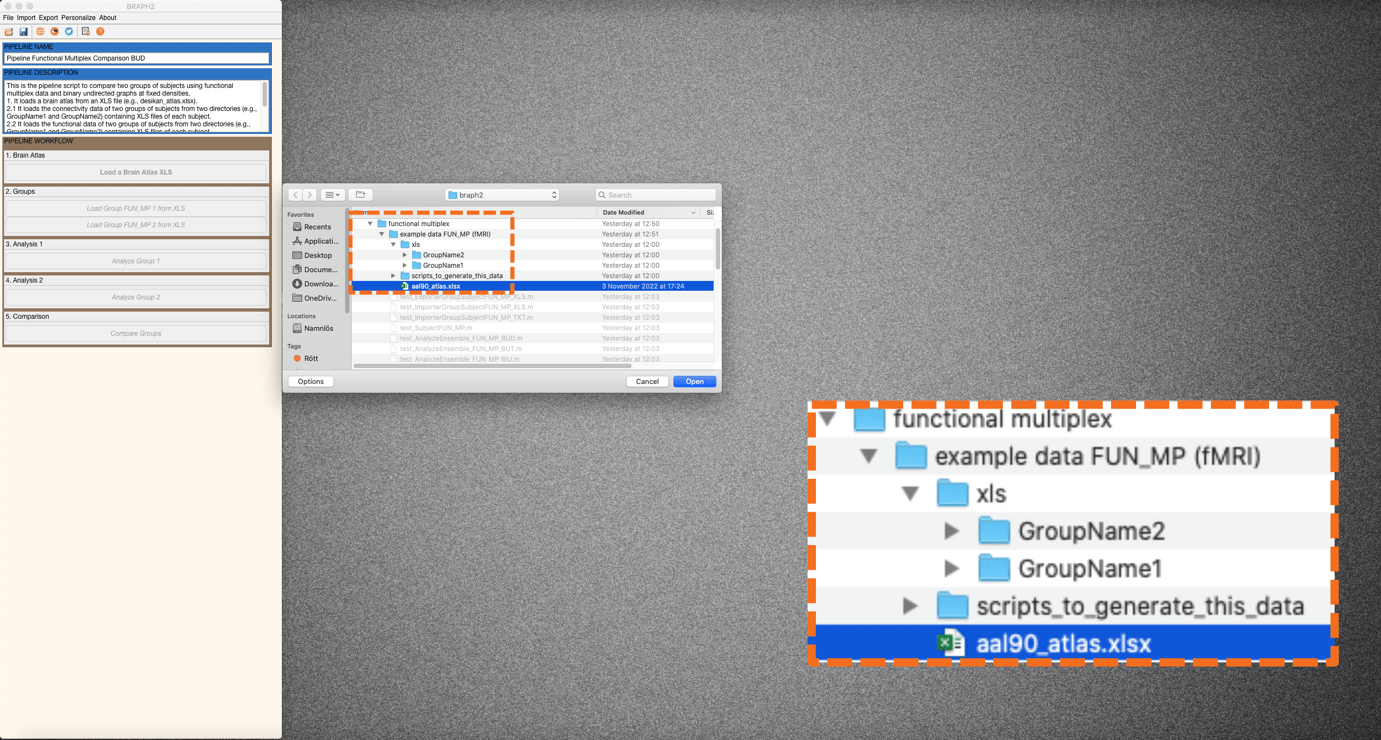The first step is to load a brain atlas from an XLS file. Press the button “Load a Brain Atlas XLS” (the only one active in the pipeline GUI, Figure 2) and then select the aal90_atlas.xlsx in the directory ./braph2/pipelines/functional multiplex/example data FUN_MP (fMRI) (Figure 3).

Finally, you can visualize the atlas by pressing “Plot the brain atlas” and play with the surface settings. Check the Brain Atlas section from the tutorial of module 1 for more information on how to control the appearance in this interface.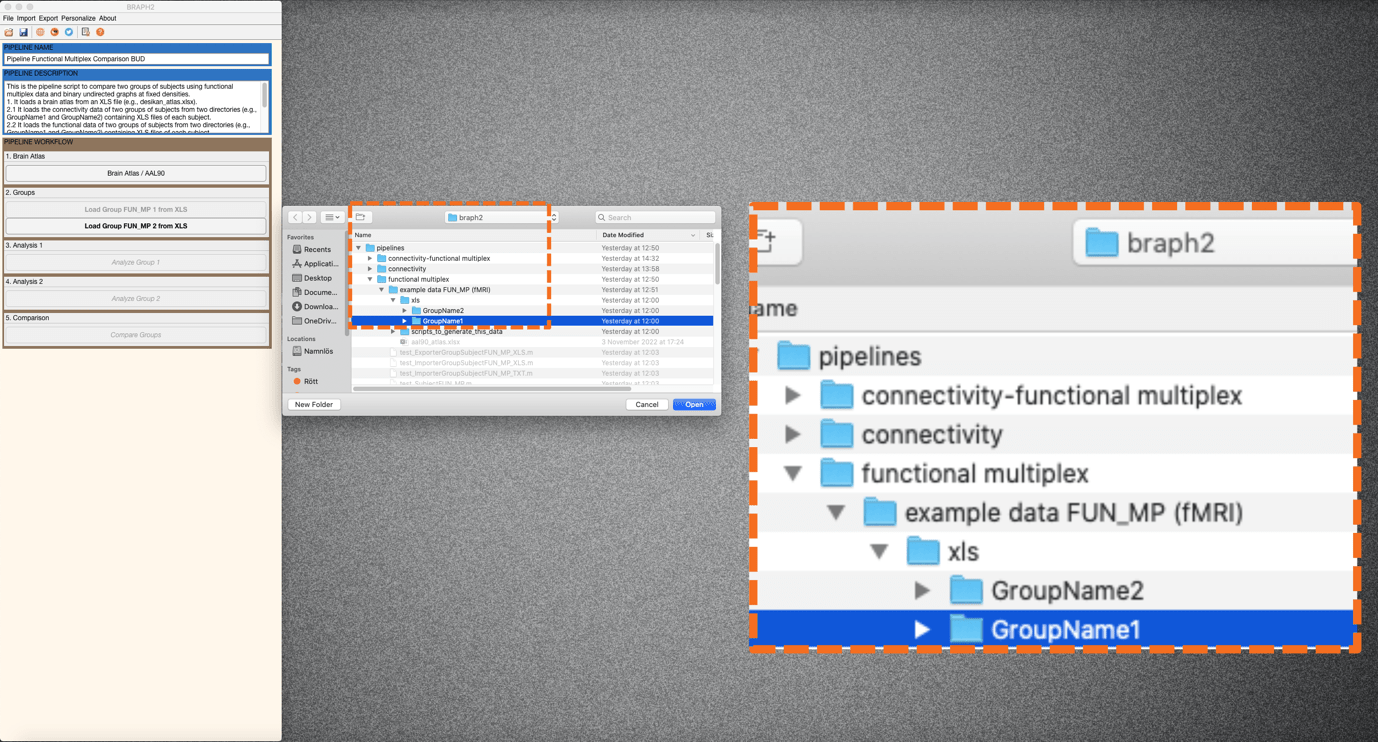Figure 4. From the pipeline GUI, first load data for group 1 (GroupName1) and then for group 2.

To load the data for the two groups you would like to compare press “Load Group FUN_MP 1 from XLS” and select the folder for subjects of Group1 which is in the directory ./braph2/pipelines/functional multiplex/example data FUN_MP (fMRI)/xls/GroupName1 (Figure 4). After, load the data for group 2 which can be found in the directory ./braph2/pipelines/functional multiplex/example data FUN_MP (fMRI)/xls/GroupName2.

• Performing the functional multiplex analysis of group 1

Analysis of the first group using functional multiplex analyses (FUN MP) based on binary undirected graphs at fixed densities (BUD).

First press “Analyze Group 1” to open the interface for the analysis (Figure 5). In this interface, you can specify the parameters for constructing the multiplex graph from the fMRI time series from each layer (in this example data, 3 layers) such as the type of correlation, and what to do with the negative weights. In the densities section, you can specify the densities to use in the analysis by changing the default ones, in this case we will use the values “[20 25 30]” or 20:5:30.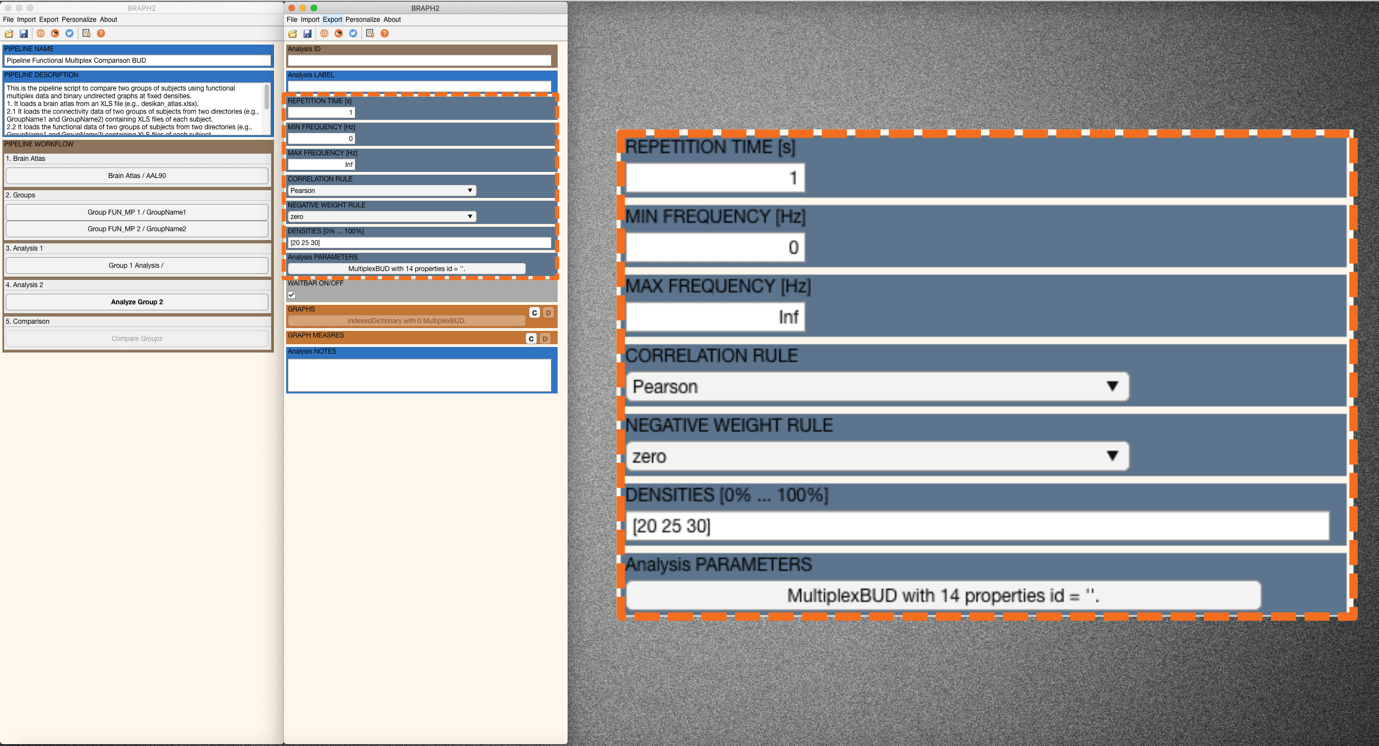Figure 5. After pressing Analyze group 1, the interface for Analysis of group 1 opens.

Then under the GRAPHS section, by pressing “C” you can check the graphs for each subject. But more interesting is to plot the average graph for the whole group; to do this go to section GRAPH MEASURES and press “C”, right-click on the left of a measure to deploy the menu seen in Figure 6 and select “Plot Graph”. We will see the weighted graph from the first layer and density, which is represented as an adjacency matrix. We can play to plot this matrix when using different densities at different layers (Figure 6).Figure 6. Plot graph. Select Mean graph, a specific density and layer.

Now it is the moment to calculate some connectivity measures for group 1. We select different measures such as the Degree, the Degree Overlap, the Degree Overlap Average, and the Edge Overlap, and calculate them by right clicking on the left-side of the measures list and press “Calculate Selected Measures” (Figure 7).Figure 7. Calculation of several measures.

When the measure is calculated, a C appears on its left, and now, again by right-clicking on the left side of the measures list and pressing “Plot Selected Measures Brain Graph”, and then checking the Measures panel in settings, we will be able to visualize the results of the nodal measures. We first visualize the Degree for group 1 (Figure 8).Figure 8. Degree brain surface visualization, first layer and first density.

In Figure 8 we see the results of Degree for layer 1 and density 20%. Because Degree is an unilayer measure, we have a measure per each layer of the network, and each density. Then we can explore the other layers, for example layer 3, keeping density at 20% (Figure 9).Figure 9. Degree brain surface visualization, third layer and first density.

Remember that blueish colors represent smaller values, and red larger. And in case you get all regions in black and same size, it means that all regions have the same value.

Then we can plot one measure that is nodal and superglobal, such as the Degree Overlap. In this case, there is one result for the network at each density. So even if you change the button layer to different layers, you will get the same result.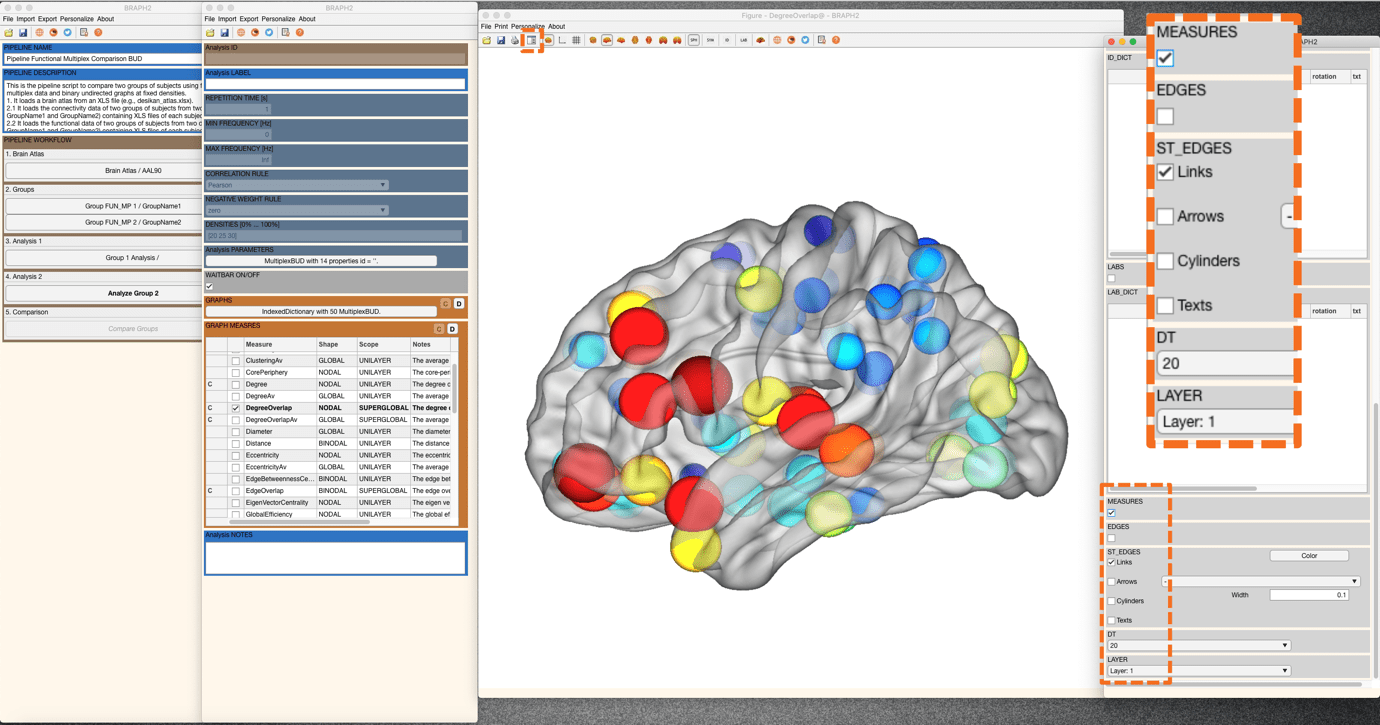Figure 10. Degree Overlap brain surface visualization, at the first density.

Remember you can also check the numerical results by pressing “Data Selected Measures” from the same menu mentioned earlier (GRAPH MEASURES) and export them to excel files. And you can plot global and binodal measures, as well as nodal, in graphs by pressing “Plot Selected Measures”.

In case we want to calculate a measure with different rules or parameters, this is done by going first to “Data Selected Measuresbefore calculating the measure. For more details, check the section Measures’ calculation with different parameters in the tutorial from Module 1.

• Performing the functional multiplex analysis of group 2

Analysis of the second group using the same parameters selected for the first group (functional multiplex analyses (FUN MP) based on binary undirected graphs at fixed densities (BUD)).

Follow the previous steps in the analysis of group 1 and explore some other measures for group 2.

• Comparing the two groups

To proceed with the group comparison press “Compare Groups” and the interface to select the comparison parameters will open. After setting the desired permutation number (recommended 1000 – 10000 permutations, although in this tutorial we use 100 permutations for time constraints), press “C” under the ENSEMBLE COMPARISONS section to open the list of measures to use to compare the groups. In this case we will compare the Multiplex Participation measure of both groups (Figure 11), by right clicking on the left of the measure table and selecting “Calculate Selected Comparisons”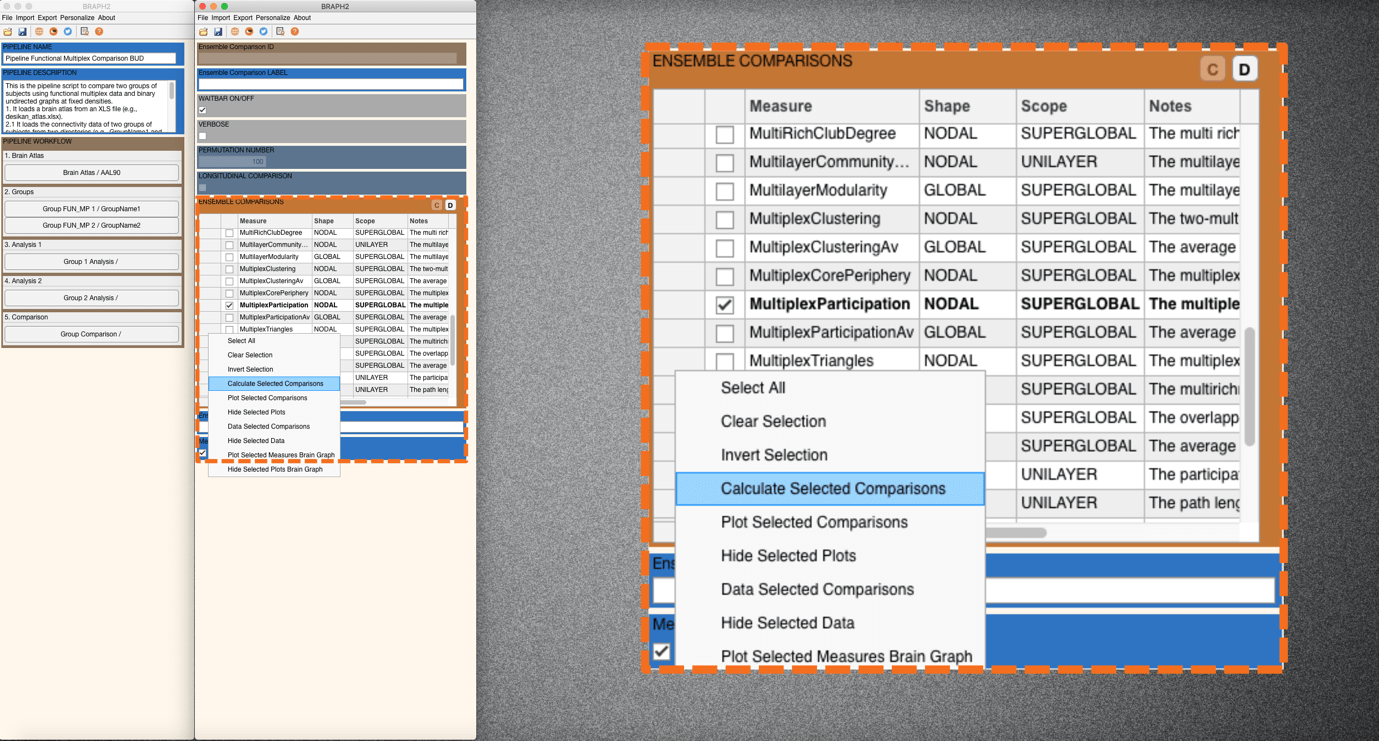Figure 11. Calculation of Group Comparison.

Depending on how many permutations you use it will take less or more time. Moreover, in connectivity and functional analyses, it takes more time than in structural, since the measure is calculated per each subject’s network from the groups, and then it is averaged to perform the comparison. If you are interested in just having average results, you can use the pipelines “Group Average”.

Once the calculations are done, you should see a C on the left of the measure to be compared. Press “Data Selected Comparison” to show the difference values between groups and the associated p-values resulting from the permutation testing. At density of 20% or 25% there are no significant differences after FDR correction at the default value of q = 0.05 (q level can be changed in the QVALUE). We plot the results from the comparison by pressing “Plot Brain Multi Graph” from the GUI containing the data tables and see the differences in multiplex participation at 25% of density when pressing MEASURES box down in the settings (Figure 12). In blue, multiplex participation is larger in group 1, and in red, is larger in group 2. If we press FDR SHOW, no region would be plotted anymore at q = 0.05.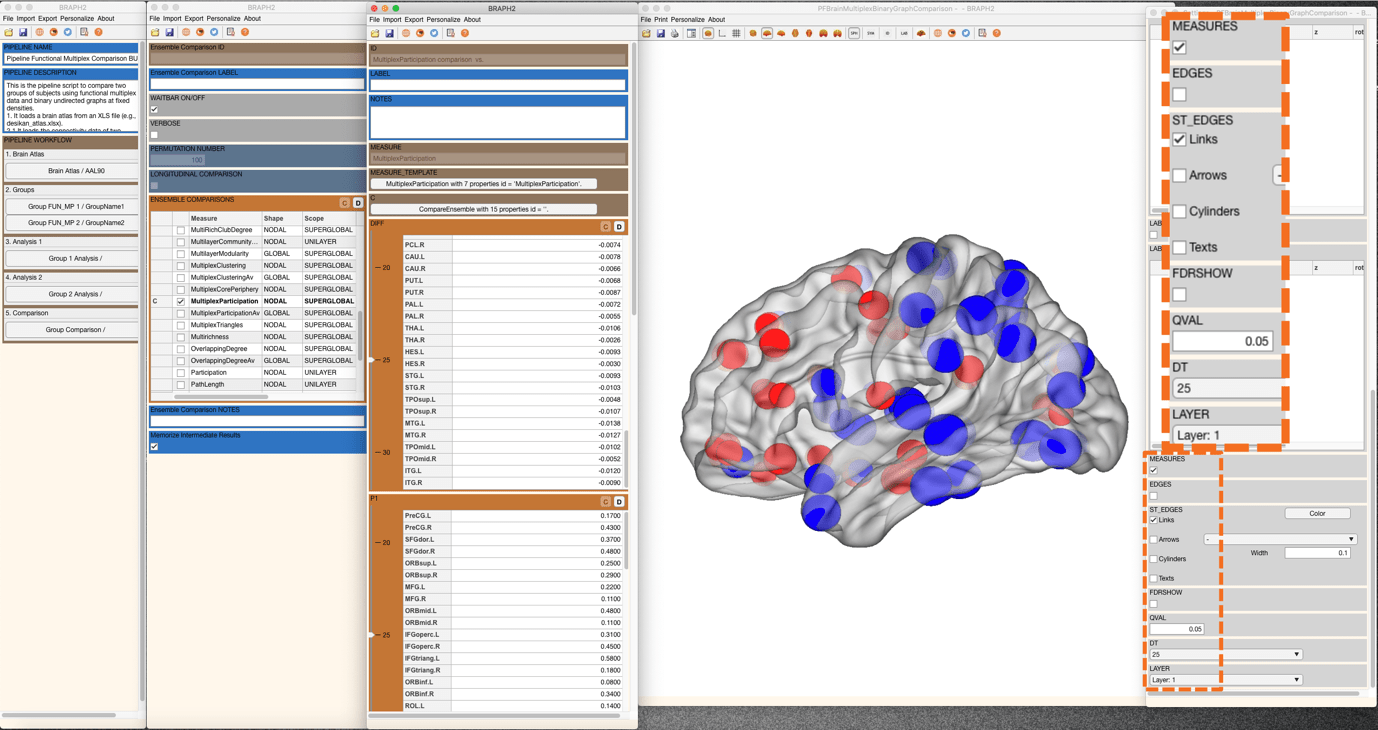Figure 12. Comparison results for density 25% of multiplex participation.

Finally, if we change the density to 30% both in the interface where we have the table results and in the settings of the brain plot, we will see that many regions have a significant difference in multiplex participation at the level of q value 0.05 (Figure 13). Moreover, all the differences are in the same direction, being group 2 the one with larger values of multiplex participation.Figure 13. Comparison results for density 30% of multiplex participation are significant after applying FDR at a q_value = 0.05.